NEET  >  Assertion & Reason Test: Electrostatic Potential & Capacitance - 1

# Assertion & Reason Test: Electrostatic Potential & Capacitance - 1

Test Description

## 15 Questions MCQ Test Physics Class 12 | Assertion & Reason Test: Electrostatic Potential & Capacitance - 1

Assertion & Reason Test: Electrostatic Potential & Capacitance - 1 for NEET 2022 is part of Physics Class 12 preparation. The Assertion & Reason Test: Electrostatic Potential & Capacitance - 1 questions and answers have been prepared according to the NEET exam syllabus.The Assertion & Reason Test: Electrostatic Potential & Capacitance - 1 MCQs are made for NEET 2022 Exam. Find important definitions, questions, notes, meanings, examples, exercises, MCQs and online tests for Assertion & Reason Test: Electrostatic Potential & Capacitance - 1 below.
Solutions of Assertion & Reason Test: Electrostatic Potential & Capacitance - 1 questions in English are available as part of our Physics Class 12 for NEET & Assertion & Reason Test: Electrostatic Potential & Capacitance - 1 solutions in Hindi for Physics Class 12 course. Download more important topics, notes, lectures and mock test series for NEET Exam by signing up for free. Attempt Assertion & Reason Test: Electrostatic Potential & Capacitance - 1 | 15 questions in 30 minutes | Mock test for NEET preparation | Free important questions MCQ to study Physics Class 12 for NEET Exam | Download free PDF with solutions
 1 Crore+ students have signed up on EduRev. Have you?
Assertion & Reason Test: Electrostatic Potential & Capacitance - 1 - Question 1

### Directions: In the following questions, A statement of Assertion (A) is followed by a statement of Reason (R). Mark the correct choice as.Assertion (A): Electric field is always normal to equipotential surfaces and along the direction of decreasing order of potential.Reason (R): Negative gradient of electric potential is electric field.

Detailed Solution for Assertion & Reason Test: Electrostatic Potential & Capacitance - 1 - Question 1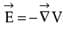So, The electric field is always perpendicular to the equipotential surface. Negative gradient of electric potential is the electric field. So, the direction of the electric field must be in the direction of the decreasing order of electric potential.

Assertion & Reason Test: Electrostatic Potential & Capacitance - 1 - Question 2

### Directions: In the following questions, A statement of Assertion (A) is followed by a statement of Reason (R). Mark the correct choice as.Assertion (A): Work done in moving a charge between any two points in a uniform electric field is independent of the path followed by the charge between these two points.Reason (R): Electrostatic forces are nonconservative.

Detailed Solution for Assertion & Reason Test: Electrostatic Potential & Capacitance - 1 - Question 2 Work done in moving a charge between any two points in a uniform electric field = charge × potential difference. So, it is independent of the path followed by the charge. Hence the assertion is true.

Electrostatic forces are conservative type. Hence, the reason is false.

Assertion & Reason Test: Electrostatic Potential & Capacitance - 1 - Question 3

### Directions: In the following questions, A statement of Assertion (A) is followed by a statement of Reason (R). Mark the correct choice as. Assertion (A): When the distance between the parallel plates of a parallel plate capacitor is halved and the dielectric constant of the dielectric used is made three times, then the capacitance becomes three times. Reason (R): Capacitance does not depend on the nature of material.

Detailed Solution for Assertion & Reason Test: Electrostatic Potential & Capacitance - 1 - Question 3

Initial capacitance =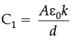Finally the capacitance =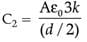So, C2 = 6C1

Hence the assertion is true.

From the expression of the capacitance, we find that capacitance depends on the area of the plates, dielectric constant and the distance between the plates. It does not depend on the nature of the material of the plates. Hence the reason is also true. But the reason cannot explain the assertion.

Assertion & Reason Test: Electrostatic Potential & Capacitance - 1 - Question 4

Directions: In the following questions, A statement of Assertion (A) is followed by a statement of Reason (R). Mark the correct choice as.

Assertion (A): Capacity of a conductor is independent of the amount of charge on it.

Reason (R): Capacitance depends on the dielectric constant of the surrounding medium, shape and size of the conductor.

Detailed Solution for Assertion & Reason Test: Electrostatic Potential & Capacitance - 1 - Question 4 C = Aε0/d

In the expression, there is no involvement of charge. So, capacitance is independent of charge. Hence the assertion is true. It depends on the permittivity of the surrounding medium and the area of the plate. So, the reason is also true. Reason explains the assertion.

Assertion & Reason Test: Electrostatic Potential & Capacitance - 1 - Question 5

Directions: These questions consist of two statements, each printed as Assertion and Reason. While answering these questions, you are required to choose any one of the following four responses.

Assertion : If the distance between parallel plates of a capacitor is halved and dielectric constant is three times, then the capacitance becomes 6 times.

Reason : Capacity of the capacitor does not depend upon the nature of the material.

Detailed Solution for Assertion & Reason Test: Electrostatic Potential & Capacitance - 1 - Question 5 We know that capacity of capacitor is directly proportional to dielectric constant and inversely proportional to distance. So, the net effect of making distance halved & making dielectric constant three times will be capacity becoming six times. As nature of the material (dielectric constant) is a factor influencing the capacity, therefore, reason is false.
Assertion & Reason Test: Electrostatic Potential & Capacitance - 1 - Question 6

Directions: These questions consist of two statements, each printed as Assertion and Reason. While answering these questions, you are required to choose any one of the following four responses.

Assertion : The total charge stored in a capacitor is zero.

Reason : The field just outside the capacitor is σ/ε0. (σ is the charge density).

Detailed Solution for Assertion & Reason Test: Electrostatic Potential & Capacitance - 1 - Question 6 Charge stored on the two plates are + Q and -Q = Q + (-Q) = 0 and hence statement A is true.The field however, outside the plates is zero. Draw a Gaussian surface ABCD as shown. The field E is uniform on faces AD and BC

∫E.ds = 0 yields E = 0

Assertion & Reason Test: Electrostatic Potential & Capacitance - 1 - Question 7

Directions: These questions consist of two statements, each printed as Assertion and Reason. While answering these questions, you are required to choose any one of the following four responses.

Assertion : Two concentric charged shells are given. The potential difference between the shells depends on the charge of the inner shell.

Reason : Potential due to charge of outer shell remains same at every point inside the sphere.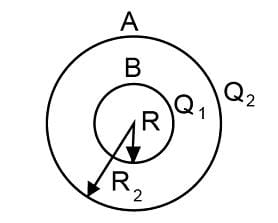Detailed Solution for Assertion & Reason Test: Electrostatic Potential & Capacitance - 1 - Question 7

For a shell of radius R with charge Q, potential on the surface or any point inside is given by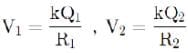Potential at inner shell is given by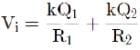Potential at outer shellPotential difference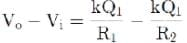Therefore, Assertion and Reason are correct and the Reason is a correct explanation of the Assertion.

Assertion & Reason Test: Electrostatic Potential & Capacitance - 1 - Question 8

Directions: These questions consist of two statements, each printed as Assertion and Reason. While answering these questions, you are required to choose any one of the following four responses.

Assertion: The potential difference between any two points in an electric field depends only on initial and final position.

Reason: Electric field is a conservative field so the work done per unit positive charge does not depend on the path followed.

Detailed Solution for Assertion & Reason Test: Electrostatic Potential & Capacitance - 1 - Question 8 Even, though field may have considerable strength, the potential difference between the two conductors of a capacitor is small because the field lines are confined within a small region of space
Assertion & Reason Test: Electrostatic Potential & Capacitance - 1 - Question 9

Directions: These questions consist of two statements, each printed as Assertion and Reason. While answering these questions, you are required to choose any one of the following four responses.

Assertion : Electric field is discontinuous across the surface of a spherical charged shell.

Reason : Electric potential is continuous across the surface of a spherical charged shell.

Detailed Solution for Assertion & Reason Test: Electrostatic Potential & Capacitance - 1 - Question 9 Electric field is zero inside the spherical charged shell and it is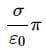outside. So it is discontinuous across the surface . Electric potential is continuous across the surface. As,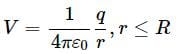Assertion & Reason Test: Electrostatic Potential & Capacitance - 1 - Question 10

Directions: These questions consist of two statements, each printed as Assertion and Reason. While answering these questions, you are required to choose any one of the following four responses.

Assertion : Two adjacent conductors of unequal dimensions, carrying the same positive charge have a potential difference between them.

Reason : The potential of a conductor depends upon the charge given to it.

Detailed Solution for Assertion & Reason Test: Electrostatic Potential & Capacitance - 1 - Question 10 Let us consider two spherical shells of radii r1 and r2 which are possessing the same positive charge Q. Therefore the potential on the surface of each conductor will be

V1 = KQ/r1

V2 = KQ/r2

Since r1 ≠ r2, V1 ≠ V2 and consequently there will be a potential difference between the two conductors.

Assertion & Reason Test: Electrostatic Potential & Capacitance - 1 - Question 11

Directions: These questions consist of two statements, each printed as Assertion and Reason. While answering these questions, you are required to choose any one of the following four responses.

Assertion : For a non-uniformly charged thin circular ring with net charge is zero, the electric field at any point on the axis of the ring is zero.

Reason : For a non-uniformly charged thin circular ring with net charge zero, the electric potential at each point on the axis of the ring is zero.

Detailed Solution for Assertion & Reason Test: Electrostatic Potential & Capacitance - 1 - Question 11 For a non-uniformly charged thin circular ring with net zero charge, electric potential at each point on its axis is zero. Hence the electric field at each point on its axis must be perpendicular to the axis. Therefore Assertion is false and Reason is true.
Assertion & Reason Test: Electrostatic Potential & Capacitance - 1 - Question 12

Directions: These questions consist of two statements, each printed as Assertion and Reason. While answering these questions, you are required to choose any one of the following four responses.

Assertion : Polar molecules have permanent dipole moments.

Reason : In polar molecules, the centres of positive and negative charges coincide even when there is no external field.

Detailed Solution for Assertion & Reason Test: Electrostatic Potential & Capacitance - 1 - Question 12 The molecules of a substance may be polar or non-polar. N a non-polar molecule, the centres of positive and negative charges conicide. This molecule has no permanent dipole moment . On the other hand, a polar molecule is one which the centres of positive and negative charges are specated, even when there is no external field. Such molecules have a permanent dipole moment.
Assertion & Reason Test: Electrostatic Potential & Capacitance - 1 - Question 13

Directions: These questions consist of two statements, each printed as Assertion and Reason. While answering these questions, you are required to choose any one of the following four responses.

Assertion : In the absence of an external electric field, the dipole moment per unit volume of a polar dielectric is zero.

Reason : The dipoles of a polar dielectric are randomly oriented.

Detailed Solution for Assertion & Reason Test: Electrostatic Potential & Capacitance - 1 - Question 13 There are polar and non polar dielectric materials. The molecules of a polar dielectric have a permanent dipole moment . However due to random orientations net dipole moment is zero. If there is no external electric field , there is no polarishation.
Assertion & Reason Test: Electrostatic Potential & Capacitance - 1 - Question 14

Directions: These questions consist of two statements, each printed as Assertion and Reason. While answering these questions, you are required to choose any one of the following four responses.

Assertion : Electric energy resides out of the spherical isolated conductor.

Reason : The electric field at any point inside the conductor is zero.

Detailed Solution for Assertion & Reason Test: Electrostatic Potential & Capacitance - 1 - Question 14 As these is no electric field inside the conductor, and so no energy inside it.
Assertion & Reason Test: Electrostatic Potential & Capacitance - 1 - Question 15

Directions: These questions consist of two statements, each printed as Assertion and Reason. While answering these questions, you are required to choose any one of the following four responses.

Assertion: Two equipotential surfaces can be orthogonal.

Reason: Electric field lines are normal to the equipotential surface.

Detailed Solution for Assertion & Reason Test: Electrostatic Potential & Capacitance - 1 - Question 15 Two equipotential surfaces never intersect each other so they cannot be orthogonal.

## Physics Class 12

157 videos|430 docs|213 tests
 Use Code STAYHOME200 and get INR 200 additional OFF Use Coupon Code
Information about Assertion & Reason Test: Electrostatic Potential & Capacitance - 1 Page
In this test you can find the Exam questions for Assertion & Reason Test: Electrostatic Potential & Capacitance - 1 solved & explained in the simplest way possible. Besides giving Questions and answers for Assertion & Reason Test: Electrostatic Potential & Capacitance - 1, EduRev gives you an ample number of Online tests for practice

## Physics Class 12

157 videos|430 docs|213 tests

### How to Prepare for NEET

Read our guide to prepare for NEET which is created by Toppers & the best Teachers